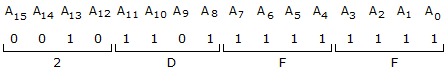# Electronics and Communication Engineering - Exam Questions Papers

1.

In the signal flow graph of figure below, the gain C/R will be :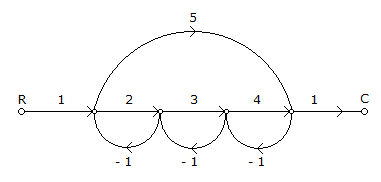A. 11/9 B. 22/15 C. 24/23 D. 44/23

Explanation:

Forward path gain

P1 = 2 x 3 x 4 ⇒ 24, P2 = 5, D1 = 1

D2 = - 3, L1 = - 2, L2 = - 3, L3 = - 4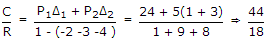Two non touching loop = L1 x L3 = - 2 x - 4 = 8

But if we take loop L4 into consideration then = 5 x -1 x - 1 x - 1 = -5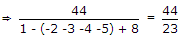.

2.

The resonant frequency of the circuit shown in figure is,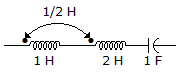A. 1/22p Hz B. 1/2p Hz C. 1/4p Hz D. 1/2p Hz

Explanation:

Leq = L1 + L2 + 2M

Leq = 1 + 2 + 2 x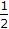= 4 H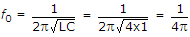.

3.

A short grounded vertical antenna has a length L which is 0.05λ at frequency f. If its radiation resistance at f is R ohms, then its radiation resistance at a frequency 2f will be

 A. R/2Ω B. RΩ C. 2RΩ D. 4RΩ

Explanation:

Rr = 80p2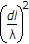Rrf2.

4.

Which of the following is a solution to the differential equation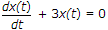?

 A. x(t) = 3e-t B. x(t) = 2e-3t C.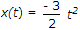D. x(t) = 3t2

Explanation:log x(t) = 3t

x(t) = K.e-3t

Thus option (B) satisfies the solution.

5.

In the circuit shown, the device connected to Y5 can have address in the range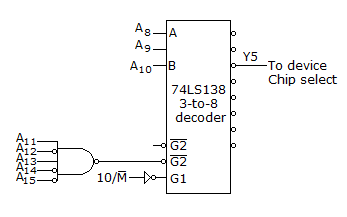A. 2000 - 20FF B. 2D00 - 2DFF C. 2E00 - 2EFF D. FD00 - FDFF